Question

# Calculate the freezing point and boiling point of a solution containing 17.4 g of naphthalene (C10H8)...

Calculate the freezing point and boiling point of a solution containing 17.4 g of naphthalene (C10H8) in 112.0 mL of benzene. Benzene has a density of 0.877 g/cm3. Part A Calculate the freezing point of a solution. (Kf(benzene)=5.12∘C/m.) Part B Calculate the boiling point of a solution. (Kb(benzene)=2.53∘C/m.)

We need at least 10 more requests to produce the answer.

0 / 10 have requested this problem solution

The more requests, the faster the answer.

All students who have requested the answer will be notified once they are available.

#### Earn Coins

Coins can be redeemed for fabulous gifts.

Similar Homework Help Questions
• ### Calculate the freezing point and boiling point of a solution containing 20.0 g of naphthalene (C_10H_8)...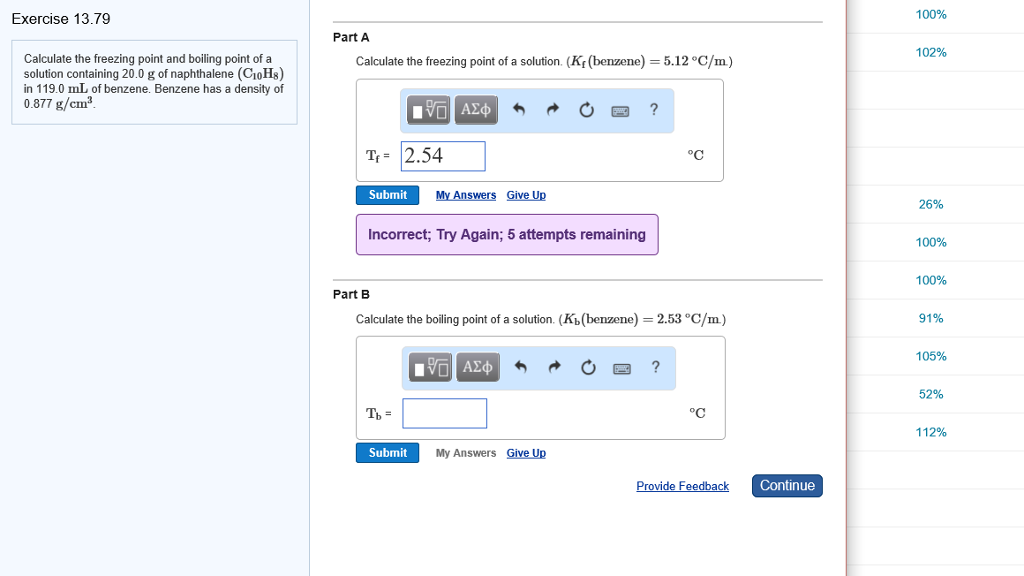Calculate the freezing point and boiling point of a solution containing 20.0 g of naphthalene (C_10H_8) in 119.0 mL of benzene. Benzene has a density of 0877 g/cm^3. Calculate the freezing point of a solution (K_f (benzene) = 5.12 degree C/m) T_f = 2.54 Calculate the boiling point of a solution. (K_b (benzene) = 2.53 degree C/m) T_b =

• ### Calculate the freezing point and boiling point of a solution containing 10.0 g of naphthalene (C10Hz)...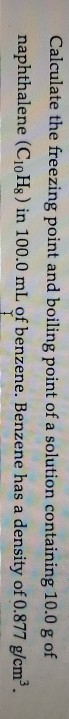Calculate the freezing point and boiling point of a solution containing 10.0 g of naphthalene (C10Hz) in 100.0 mL of benzene. Benzene has a density of 0.877 g/cm².

• ### Determine the freezing point of a solution that contains 65.4 g of naphthalene (C10H8) dissolved i...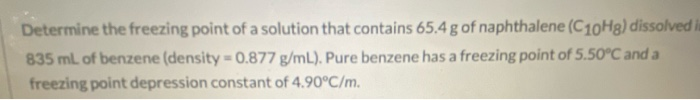Determine the freezing point of a solution that contains 65.4 g of naphthalene (C10H8) dissolved i 835 mL of benzene (density -0.877 g/mL). Pure benzene has a freezing point of 5.50°C and a freezing point depression constant of 4.90°C/m.

• ### MISSED THIS? Read Section 14.6 (Pages 601 - 613); Watch KCV 14.6, IWE 14.9. Calculate the...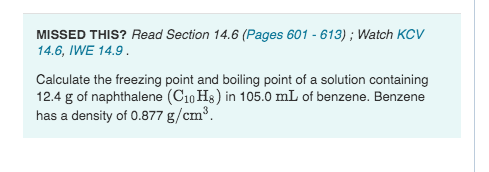MISSED THIS? Read Section 14.6 (Pages 601 - 613); Watch KCV 14.6, IWE 14.9. Calculate the freezing point and boiling point of a solution containing 12.4 g of naphthalene (C10H8) in 105.0 mL of benzene. Benzene has a density of 0.877 g/cm Part A Calculate the freezing point of a solution. (K: (benzene) = 5.12 °C/m.) Express your answer in degrees Celsius to one decimal place. O ASC O O ? Ts = Submit Request Answer Part B Calculate the...

• ### What are the boiling point and freezing point of a 4.97 m solution of naphthalene in...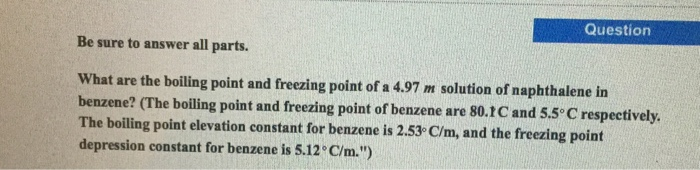What are the boiling point and freezing point of a 4.97 m solution of naphthalene in benzene? (The boiling point and freezing point of benzene are 80.1 C and 55 degree C respectively. The boiling point elevation constant for benzene is 2.53 C/m, and the freezing point depression constant for benzene is 5.12 degree C/m.'')

• ### What are the boiling point and freezing point of a 4.97 m solution of naphthalene in...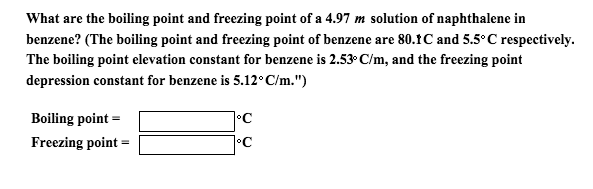What are the boiling point and freezing point of a 4.97 m solution of naphthalene in benzene? (The boiling point and freezing point of benzene are 80.IC and 5.5 degree C respectively. The boiling point elevation constant for benzene is 2.53 degree C/m, and the freezing point depression constant for benzene is 5.12 degree C/m.) Boiling point = degree C Freezing point = degree C

• ### 27) Determine the freezing point of a solution that contains 78.8 g of naphthalene (C10H8, molar...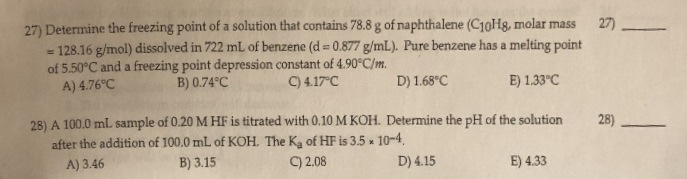27) Determine the freezing point of a solution that contains 78.8 g of naphthalene (C10H8, molar mass = 128.16 g/mol) dissolved in 722 mL of benzene (d = 0.877 g/mL). Pure benzene has a melting point of 5.50°C and a freezing point depression constant of 4.90°C/m. A) 4.76°C 27) C) 4.17°C E) 1.33°C D) 1.68°C B) 0.74°C 28) A 100,0 mL sample of 0.20 M HF is titrated with 0.10 M KOH. Determine the pH of the solution after the...

• ### 1)The freezing point change for a solution containing naphthalene (a nonvolatile, nonionizing compound) in 250. g...

1)The freezing point change for a solution containing naphthalene (a nonvolatile, nonionizing compound) in 250. g of liquid benzene is 10.2°C. What is the amount (moles) of naphthalene in the solution? (Kf = 5.12°C/m for benzene) 2)Acidic acid is a strong acid. a)True b)False

• ### Calculate the freezing point and boiling point of a solution containing 8.40 g of ethylene glycol...

Calculate the freezing point and boiling point of a solution containing 8.40 g of ethylene glycol (C2H6O2) in 88.1 mL of ethanol. Ethanol has a density of 0.789 g/cm3. Calculate the freezing point of the solution. Calculate the boiling point of the solution.

• ### Be sure to answer all parts. What are the boiling point and freezing point of a...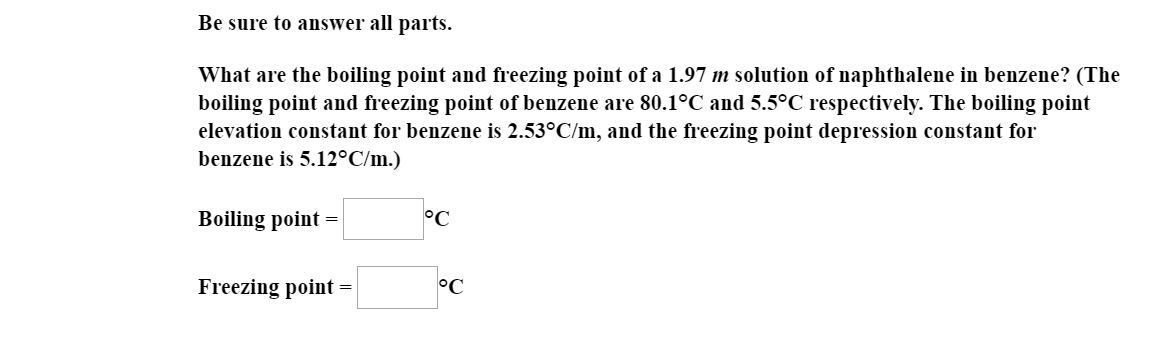Be sure to answer all parts. What are the boiling point and freezing point of a 1.97 m solution of naphthalene in benzene? (The boiling point and freezing point of benzene are 80.1°C and 5.5°C respectively. The boiling point elevation constant for benzene is 2.53°C/m, and the freezing point depression constant for benzene is 5.12°C/m.) Boiling point = Freezing point =